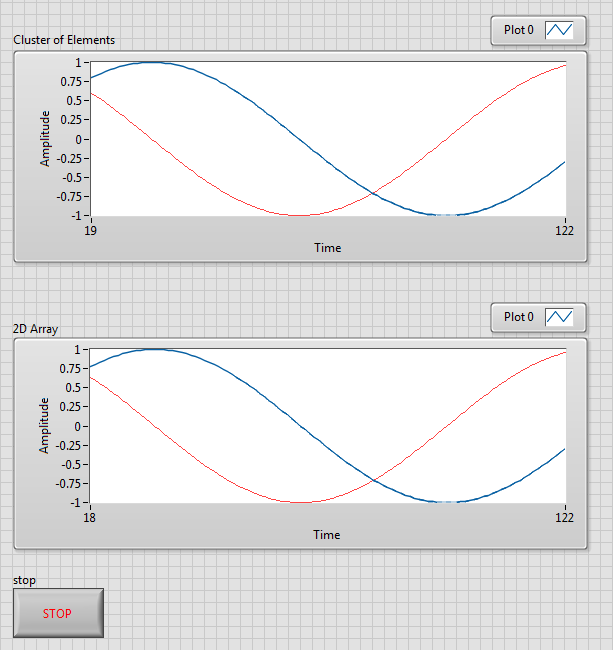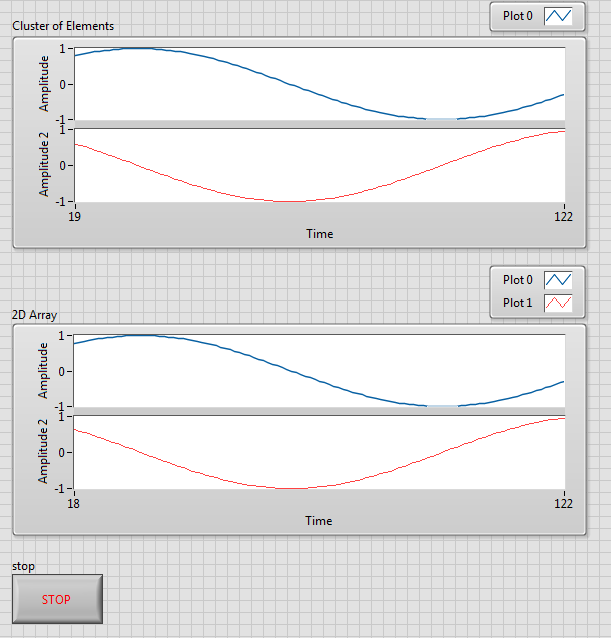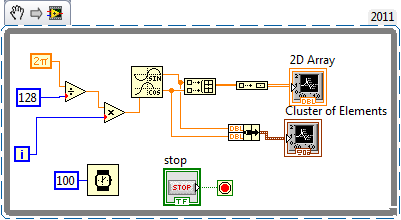# Example Program Drafts

cancel
Showing results for
Did you mean:

## Viewing Multiple Waveforms as Separate Plots on the Same Waveform Chart

• ### Example Code

Overview

This program takes data in the form of a two-dimensional array and a cluster of elements and displays multiple plots on a single waveform charts. With this example stacked plots can also be created.

Description

This program generates a sine and cosine waves and plots them to a waveform chart. This is done by creating a cluster of elements as well as a 2D array. Using both data types in 2 separate charts allows the user to better understand the different steps necessary to create stacked plots for each chart.

Steps to Implement or Execute Code

1. Open the Multiple Plots VI.
2. Run the code and see how you can successfully have multiple plots on a single chart.
3. Create stacked plots.
1. On the cluster of elements chart right click and select "Stack Plots".
2. Right click on the 2D array chart and go to visible items and make the plot legend visible.
3. Resize the 2D array chart plot legend to contain two plots.
4. Right click on the 2D array chart and select stack plots.

Requirements

Software

LabVIEW 2011

HardwareFigure 1: This figure displays how multiple plots can be displayed on one chartFigure 2: This figure shows stacked plots which is a different way to display multiple plots on the same chart. Notice the 2D Array chart has to have both plots in the plot legend.Matt P.
Applications Engineer
National Instruments
Contributors